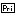#Priority Encoder

 Library: Plexers Introduced: 2.3.0 Appearance:## Behavior

The component has a number of inputs on its west edge, with the first labeled 0 and the other numbered from there. The component determines the indices of the inputs whose values are 1, and it emits the highest index. For example, if inputs 0, 2, 5, and 6 are all 1, then the priority encoder emits a value of 110. If no inputs are 1, or if the component is disabled, then the output of the priority encoder is floating.

The priority encoder is designed so that a number of encoders can be daisy-chained to accommodate additional inputs. In particular, the component includes an enable input and an enable output. Whenever the enable input is 0, the component is disabled, and the output will be all floating bits. The enable output is 1 whenever the component is enabled and none of the indexed inputs are 1. Thus, you can take two priority encoders and connect the enable output of the first to the enable input of the second: If any of the indexed inputs to the first are 1, then the second will be disabled and so its output will be all floating. But if none of the first's indexed inputs are 1, then its output will be all-floating bits, and the second priority encoder will be enabled and it will identify the highest-priority input with a 1.

An additional output of the priority encoder is 1 whenever the priority encoder is enabled and finds a 1 on one of the indexed inputs. When chaining priority encoders together, this output can be used to identify which of the encoders was triggered.

## Pins

West edge, variable number (inputs, bit width 1)
Input values, indexed from 0 at the top/west end of the edge.
East edge, upper pin (output, bit width matches Select Bits attribute)
Output: the highest index among those inputs whose value is 1 - or all floating bits if no inputs are 0 or if the component is disabled via the Enable In input.
East edge, lower pin (output, bit width 1)
Group Signal: 1 if the component is enabled and at least one indexed input has a value of 1; otherwise this output is 0.
South edge (input, bit width 1)
Enable In: if 0, the component is disabled; otherwise the component is enabled.
North edge (output, bit width 1)
Enable Out: 1 if this component is enabled and none of the indexed inputs are 1; otherwise the output is 0.

## Attributes

Facing
The direction of the component (its output relative to its input).
Select Bits
The bit width of the component's primary output. The number of indexed inputs to the priority encoder will be 2selectBits.

None.

## Text Tool Behavior

None.

Back to Library Reference